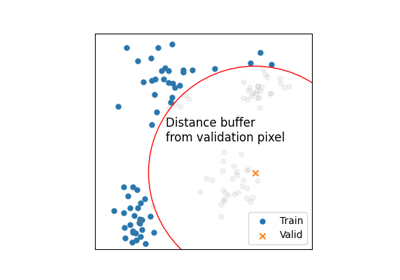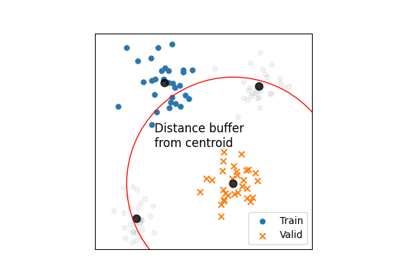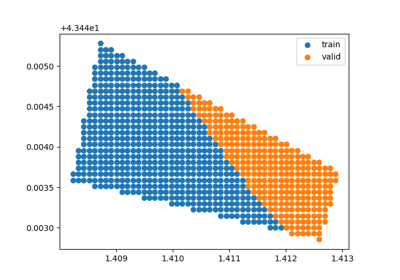# get_distance_matrix¶

`museotoolbox.processing.``get_distance_matrix`(in_image, in_vector, field=False, verbose=False)[source]

Return for each pixel, the distance one-to-one to the other pixels listed in the vector.

Parameters
• in_image (str.) – Path of the raster file where the vector file will be rasterize.

• in_vector (str.) – Path of the vector file to rasterize.

• field (str or False, optional (default=False)) – Name of the vector field to extract the value (must be float or integer).

Returns

• distance_matrix (array of shape (nSamples,nSamples))

• label (array of shape (nSamples))

## Examples using `museotoolbox.processing.get_distance_matrix`¶